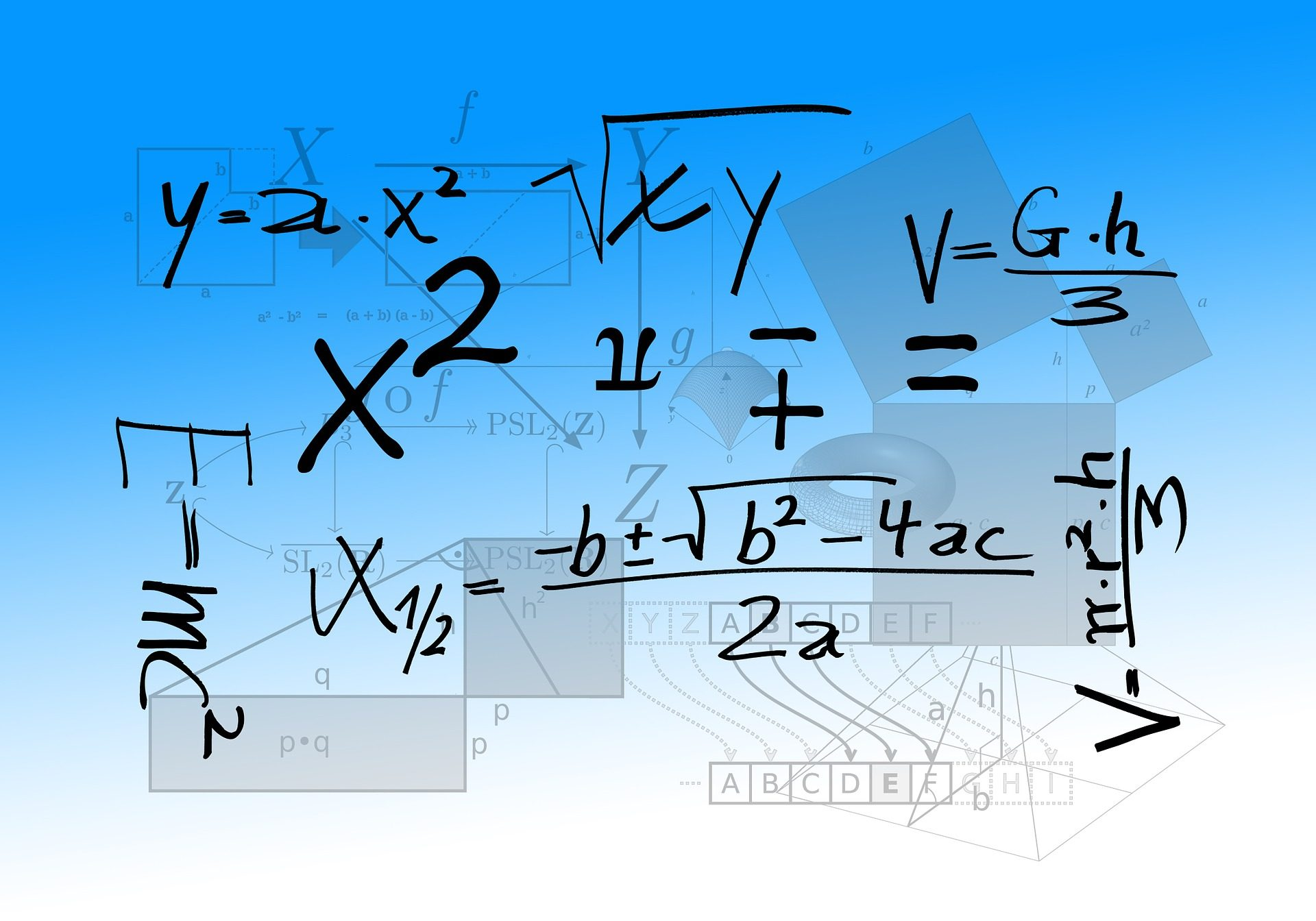# Tutorial Five: How Einstein finds an averageNow that you’ve been introduced to averages and Doppler equations, let’s combine these two ideas. This will also give us an opportunity to start looking at Einstein’s theory of relativity. We’ll begin with a few questions and answers:

1. Do you need relativity theory to find the average of two numbers?
Answer: No, you do not need relativity theory to find an average.
2. In Section 3 of Einstein’s 1905 derivation, what is the meaning vx’ / (c2 – v2)?

To begin demystifying relativity theory, let’s find the average Doppler wavelength equation. Recapping Tutorial Four, the Doppler wavelength equations for an approach light source is  x’ / (1 + v/c) and a receding light source is  x’ / (1 – v/c), where x’ is the original wavelength, v is the velocity of the light source, and c is the propagation speed of the light wave. Previously we used w, instead of c, where it represented the speed of sound through air. The average Doppler equation, ξ, is easily found using the addition mean equation ξ  = (U1 + U2) / 2, which produces:

ξ = x’ / (1 – v2 / c2)

We found this equation without using relativity theory. What’s important to notice is that this exact equation appears in Einstein’s paper! What’s also interesting is that Einstein does not realize that he arrives at an average, probably because he does not use the addition mean equation.

Let’s look at what Einstein does. First, begin by dividing the receding and approaching Doppler equations by c. Don’t worry, we’ll multiply it back in shortly. These equations are x’ / (c – v) and x’ / (c + v).

The average, τ, of two numbers is also found using the less–familiar subtraction mean equation τ  = U1 – (U1 – U2) / 2, which does not use the addition operator.  In the subtraction mean equation, the expression (U1 – U2) / 2 is called the half–difference. Grounded in classical mechanics, Einstein says that the time for a ray of light to travel in one direction is x’ / (c – v) and in the reverse direction is x’ / (c + v). The half–difference of these expressions is:

vx’ / (c2 – v2)

This expression vx’ / (c– v2) is not a non–specific “adjustment to time” as it is commonly explained. It is the half–difference, which when subtracted from the larger operand, x’ / (c – v), produces the average of the two expressions. Einstein performs this subtraction in Section 3 of his paper, which he immediately multiplies by c to arrive at:

ξ = x’ / (1 – v2 / c2)

Although his approach is complicated by his use of a function and a partial differential equation, Einstein finds the average ξ using the non–obvious subtraction mean equation rather than with the familiar addition mean equation. Failing to recognize ξ as an average, Einstein will interpret it to represent the x-axis coordinate of a space–time point.

Summarizing, we’ve shown that:

1. You don’t need relativity to find an average.
2. Einstein’s ξ equation (prior to his final adjustments) is identical to the average Doppler equation.
3. The expression vx’ / (c– v2is the half–difference, not a non–specific time adjustment.

This is only the beginning of our look at Einstein’s theory, which we’ll come back to in later tutorials.

—–

DISRUPTIVE is the #1 New Release in Physics/Relativity and Physics/Mechanics on Amazon.com.* Available now at Amazon.com, BarnesAndNoble.com, and other booksellers!